# Physics - Spherical Lenses

## Introduction

• A transparent material (normally glass) bound by two surfaces, of which one or both surfaces are spherical, is known as "spherical lens."## Convex Lens

• A lens may have two spherical surfaces, bulging outwards (as shown in the image given below), is known as convex lens or a double convex lens.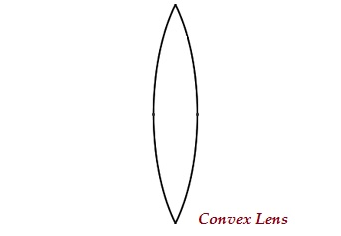• The middle part of this lens is bulged (thicker) and at the both ends, it is narrow.

• Convex lens converges the light rays; therefore, it is also known as converging lens.

## Concave Lens

• A lens may have two spherical surfaces, curved inwards (as shown in the image given below), is known as concave lens or a double concave lens.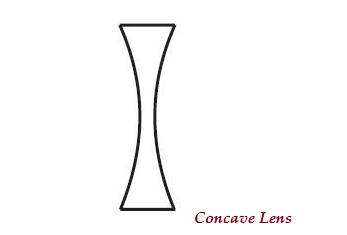• The middle part of this lens is narrow (curved inwards) and the both the edges are thicker.

• Concave lens diverges the light rays; therefore, it is also known as diverging lens.

• A lens, either a concave or a convex, has two spherical surfaces and each of these surfaces forms a part of the sphere. The centers of these spheres are known as centers of curvature, represented by English letter ‘C.’

• As there are two centers of curvature, therefore, represented as ‘C1’ and ‘C2.’

• An imaginary straight line, passing through both the centers of curvature of a lens, is known as principal axis.

• Optical center is the central point of a lens. It is represented by ‘O.’

• An aperture is the actual diameter of the circular outline of a spherical lens.

• Principal focus of lens is represented by ‘F.’

• A lens has usually two foci represented as F1 and F2.

• Focal length is the distance between the principal focus and the optical center of a lens. It is represented by ‘f.’

• The following table illustrates, the nature and position of images formed by a convex lens −

Position of Object Position of Image Size of Image Nature of Image Image
At infinity At the focus F2 Highly diminished, pointsized Real and inverted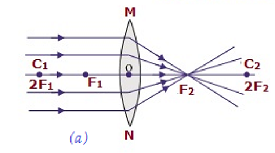Beyond 2F1 B/w F2 and 2F2 Diminished Real and inverted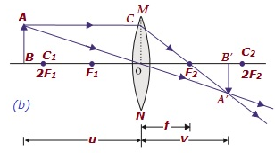At 2F1 At 2F2 Same size Real and inverted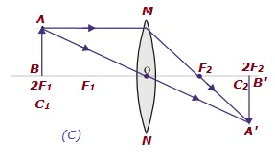B/w F1 & 2F1 Beyond 2F2 Enlarged Real and inverted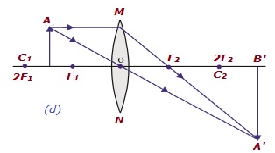At focus F1 At infinity Infinitely large or highly enlarged Real & inverte d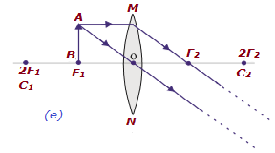B/w focus F1 & optical center O On the same side of the lens as the object Enlarged Virtual and erect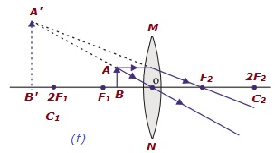• The following table illustrates, the nature and position of images formed by a concave lens −

Position of Object Position of Image Relative Size of Image Nature of Image Image
At infinity At the focus F1 Highly diminishe d, pointsized Virtual and erect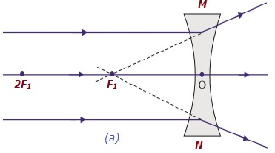B/w infinity & optical center O of the lens B/w F1 & optical center O Diminishe d Virtual and erect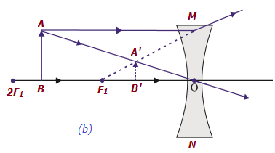## Lens Formula

• The formula is expressed as −

• $$\frac{1}{v} - \frac{1}{u} = \frac{1}{f}$$

• Lens formula expresses the relationships among the object-distance (i.e. u), image-distance (i.e. v), and focal length (i.e. f) of a lens.